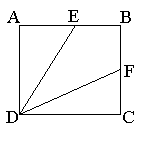# Problem Solving MCQ Quiz - 1

## 10 Questions MCQ Test Quantitative Aptitude for Banking Preparation | Problem Solving MCQ Quiz - 1

Description
Attempt Problem Solving MCQ Quiz - 1 | 10 questions in 10 minutes | Mock test for UPSC preparation | Free important questions MCQ to study Quantitative Aptitude for Banking Preparation for UPSC Exam | Download free PDF with solutions
QUESTION: 1

### Jo's collection contains US, Indian and British stamps. If the ratio of US to Indian stamps is 5 to 2 and the ratio of Indian to British stamps is 5 to 1, what is the ratio of US to British stamps?

Solution:

Indian stamps are common to both ratios. Multiply both ratios by factors such that the Indian stamps are represented by the same number.
US : Indian = 5 : 2, and Indian : British = 5 : 1. Multiply the first by 5, and the second by 2.
Now US : Indian = 25 : 10, and Indian : British = 10 : 2
Hence the two ratios can be combined and US : British = 25 : 2

QUESTION: 2

### A 3 by 4 rectangle is inscribed in circle. What is the circumference of the circle?

Solution:

Draw the diagram. The diagonal of the rectangle is the diameter of the circle. The diagonal is the hypotenuse of a 3, 4, 5 triangle, and is therefore, 5.
Circumference = π.diameter = 5π

QUESTION: 3

### Two sets of 4 consecutive positive integers have exactly one integer in common. The sum of the integers in the set with greater numbers is how much greater than the sum of the integers in the other set?

Solution:

If two sets of four consecutive integers have one integer in common, the total in the combined set is 7., and we can write the sets as
n + (n + 1) + (n + 2) + (n + 3 ) and
(n + 3) + (n + 4) + (n + 5) + (n + 6)
Note that each term in the second set is 3 more than the equivalent term in the first set. Since there are four terms the total of the differences will be 4 x 3 = 12

QUESTION: 4ABCD is a square of side 3, and E and F are the mid points of sides AB and BC respectively. What is the area of the quadrilateral EBFD ?

Solution:

(Total area of square - sum of the areas of triangles ADE and DCF) will give the area of the quadrilateral
9 - (2 x ½ x 3 x 1.5) = 4.5

QUESTION: 5

If n ≠ 0, which of the following must be greater than n?

I. 2n
II. n2
III. 2 - n

Solution:

Remember that n could be positive negative or a fraction. Try out a few cases:
In case I, if n is -1, then 2n is less than n.
In case II, if n is a fraction such as ½ then n2 will be less than n.
In case III, if n is 2, then 2-n = 0, which is less than n.
Therefore, none of the choices must be greater than n

QUESTION: 6

n and p are integers greater than 5n is the square of a number 75np is the cube of a number.The smallest value for n + p is

Solution:

The smallest value for n such that 5n is a square is 5.
75np can now be written as 75 x 5 x p.
This gives prime factors.... 3 x 5 x 5 x 5 x p
To make the expression a perfect cube, p will have to have factors 3 x 3 , and hence p =9
n + p = 5 + 9 = 14

QUESTION: 7

The distance from town A to town B is five miles. C is six miles from B. Which of the following could be the distance from A to C?

I. 11
II. 1
III. 7

Solution:

Do not assume that AB and C are on a straight line. Make a diagram with A and B marked 5 miles apart. Draw a circle centered on B, with radius 6. C could be anywhere on this circle. The minimum distance will be 1, and maximum 11, but anywhere in between is possible.

QUESTION: 8

For how many integer values of n will the value of the expression 4n + 7 be an integer greater than 1 and less than 200?

Solution:

1 < 4n + 7 < 200
n can be 0, or -1
n cannot be -2 or any other negative integer or the expression 4n + 7 will be less than1.
The largest value for n will be an integer < (200 - 7) /4
193/4 = 48.25, hence 48
The number of integers between -1 and 48 inclusive is 50

QUESTION: 9

12 litres of water are poured into an aquarium of dimensions 50cm length, 30cm breadth, and 40cm height. How high (in cm) will the water rise?(1 litre = 1000cm3)

Solution:

Total volume of water = 12 liters = 12 x 1000 cm3
The base of the aquarium is 50 x 30 = 1500cm2
Base of tank x height of water = volume of water
1500 x height = 12000; height = 12000 / 1500 = 8

QUESTION: 10

Six years ago Anita was P times as old as Ben was. If Anita is now 17 years old, how old is Ben now in terms of P ?

Solution:

Let Ben's age now be B
Anita's age now is A.
(A - 6) = P(B - 6)
But A is 17 and therefore 11 = P(B - 6)
11/P = B-6
(11/P) + 6 = BUse Code STAYHOME200 and get INR 200 additional OFF Use Coupon Code